##帐号 自动登录 找回密码 密码 立即注册
 搜索

# [已解决]菜鸡求帮助！！枚举暴力的一道水题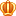发表于 2019-2-8 10:15:16 | 显示全部楼层 |阅读模式
10鱼币
 题目描述 平面上有n个点,现在你需要建造两条路，一条是斜率为1, 另一条斜率为-1 你的任务是让这两条路经过尽可能多的点 求最多经过几个点 输入描述: 第一行输入一个整数N表示点的个数 第二行输入N个数表示X坐标 第三行输入N个数表示Y坐标 1<=N<=1000 ,0<=x,y<=999 输出描述: 输出一个整数 示例1 输入 4 1 4 4 5 3 0 2 3 输出 4 说明 (1,3) (4,0) (4,2) (5,3)四个点都可以被经过 贴上我的垃圾代码 #include int main() {         int N,i,j;         int str1;         int str2;         int flag;// 判断斜率是否为1和-1         int count = 0;//计算出经过的点                 scanf("%d", &N);                 for(i = 0; i < N; i++)         {                 scanf("%d", &str1[i]);//输入x的值         }                 for(j = 0; j < N; j++)         {                 scanf("%d", &str2[j]);//输入y的值         }                 for(i = 0; i < N; i++)         {                 for(j = 0; j < N; j++)                 {                         flag = (str1[i+j] - str1[i])/(str2[i+j] - str2[i]);//算斜率                         while(flag == 1 || flag == -1)                         {                                 count+=2;                                }                 }         }         printf("%d", count);                }复制代码 代码我肯定写的有问题 但本人太菜太菜 不会写这个题目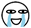最佳答案前路 2019-2-8 10:15:17 本帖最后由 前路 于 2019-2-20 00:42 编辑 以下回复为个人拙见：（诚心接受指正，不接受撕逼） 解题思路为： 利用暴力枚举列出是符合斜率的所有直线； 然后再在每条直线中枚举出所有的点； 最后进行判断输出； 知识和注意点： 1，直线公式：y=x+b； 2，x，y>0; 3，x,b都在变化，b变化时代表直线改变，x变化是代表直线上的点的变化 过程： 1，对输入格式进行分析，调试； 2，采用二维数组将测试点凸显出来用于后面的点的判断； 3，用循环for (i = 0; i < 100; i++){去枚举区域内所有符合斜率的直线 4，用循环 for (temp_x = 0; temp_x < i+1; temp_x++)去枚举每条直线的所有点 5，判断该条直线是否存在测试点 6，由于需要记录测试点存在于每条直线的情况，所以用result数组来记录每条直线上存在测试点的个数 7，遍历result数组，找出最多测试点的直线，用变量Slope_Result_1和Slope_Result_1记录最大值 8，计算总和输出结果 总结： 刚开始我的思路与楼主思路相同，但是后面发现这种思路是判断所有符合斜率的点，并不是判断所有符合斜率的同一直线上的点； .best_avatar img{width: 30px;height: 30px;border-radius: 30px;}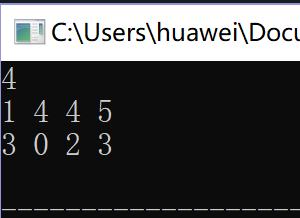### 最佳答案发表于 2019-2-8 10:15:17 | 显示全部楼层    本楼为最佳答案
 .pct{min-height:100px;}本帖最后由 前路 于 2019-2-20 00:42 编辑 以下回复为个人拙见：（诚心接受指正，不接受撕逼） 解题思路为： 利用暴力枚举列出是符合斜率的所有直线； 然后再在每条直线中枚举出所有的点； 最后进行判断输出； 知识和注意点： 1，直线公式：y=x+b； 2，x，y>0; 3，x,b都在变化，b变化时代表直线改变，x变化是代表直线上的点的变化 过程： 1，对输入格式进行分析，调试； 2，采用二维数组将测试点凸显出来用于后面的点的判断； 3，用循环for (i = 0; i < 100; i++){去枚举区域内所有符合斜率的直线 4，用循环 for (temp_x = 0; temp_x < i+1; temp_x++)去枚举每条直线的所有点 5，判断该条直线是否存在测试点 6，由于需要记录测试点存在于每条直线的情况，所以用result数组来记录每条直线上存在测试点的个数 7，遍历result数组，找出最多测试点的直线，用变量Slope_Result_1和Slope_Result_1记录最大值 8，计算总和输出结果 总结： 刚开始我的思路与楼主思路相同，但是后面发现这种思路是判断所有符合斜率的点，并不是判断所有符合斜率的同一直线上的点；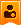楼主| 发表于 2019-2-8 10:48:19 | 显示全部楼层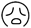大佬们 请看看我的代码吧 应该怎么改呢发表于 2019-2-8 12:31:03 | 显示全部楼层
 本帖最后由 枫还 于 2019-2-8 12:32 编辑 #include int main() {         int N,i,j;         int str1;         int str2;         int flag;// 判断斜率是否为1和-1         int count = 0;//计算出经过的点                  scanf("%d", &N);                  for(i = 0; i < N; i++)         {                 scanf("%d", &str1[i]);//输入x的值         }                  for(j = 0; j < N; j++)         {                 scanf("%d", &str2[j]);//输入y的值         }                  for(i = 0; i < N; i++)         {                 for(j = 0; j < N; j++)                 {                                 if(str1[i+j] - str1[i] != 0) {                         flag = (str2[i+j] - str2[i])/(str1[i+j] - str1[i]);//算斜率                         if(flag == 1 || flag == -1)                         {                                 count+=2;                                 }                             }                 }         }         printf("%d", count);                  }复制代码 首先判断分母不为0，用y的差去除以x的差 为什么用while？用if啊楼主| 发表于 2019-2-8 13:04:44 | 显示全部楼层
 枫还 发表于 2019-2-8 12:31 首先判断分母不为0，用y的差去除以x的差 为什么用while？用if啊 嗯嗯 这个地方要if 但是改了后的代码还是wa了 只通过了这一个样例 我想了一下这题 我没有考虑到 点可能会重复的情况 大佬可以帮忙想想这个代码要怎么改吗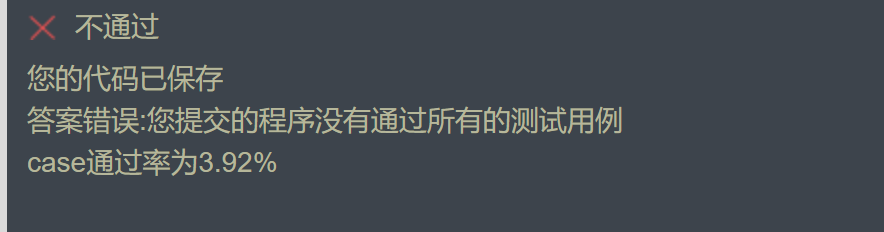楼主| 发表于 2019-2-8 17:05:21 | 显示全部楼层
 hjwwwwww 发表于 2019-2-8 13:04 嗯嗯 这个地方要if 但是改了后的代码还是wa了 只通过了这一个样例 我想了一下这题 我没有考虑到 点可能 ... @BngThea发表于 2019-2-8 18:41:00 From FishC Mobile | 显示全部楼层
 我贴出我不完善的代码。欢迎指正发表于 2019-2-8 18:41:46 From FishC Mobile | 显示全部楼层
 本帖最后由 记忆的欠片 于 2019-2-8 20:07 编辑 代码楼下发表于 2019-2-8 18:45:15 From FishC Mobile | 显示全部楼层
 #include int main(){        int N, i, j, y;        int temp = 0;        int tem = 0;        int result = {0};        int row, column;        int mat = {0};        printf("point count:");        scanf("%d", &N);        for (i = 0; i < N; i++)        {                printf("x%d:", i+1);                scanf("%d", &row[i]);        }        for (j = 0; j < N; j++)        {                printf("y%d:", j+1);                scanf("%d", &column[j]);        }        for (i = 0; i < N; i++)        {                mat[row[i]][column[i]] = 1;        }        for (j = 0; j < 100; j++)        {                for (int z = 0; z < j+1; z++)                {                        y = (-1) * z + j;                        if (mat[z][y])                        {                                result[j]++;                        }                 }        }                for (i = 0; i < sizeof(result) / sizeof(int); i++)        {                if (result[i] > temp)                {                        temp = result[i];                }                result[i] = 0;        }                for (i = 100, j = 0; i >= 0; i--, j++)        {                for (int z = i, x = 0; x < j + 1; x++, z++)                {                        y = z - i;                        if (mat[z][y])                        {                                result[j]++;                        }                }        }        for (i = 0; i < (sizeof(result) / sizeof(int)); i++)        {                if (result[i] > tem)                {                        tem = result[i];                }        }        printf("result = %d\n", temp + tem - 1);        return 0; }楼主| 发表于 2019-2-9 07:23:11 | 显示全部楼层
 记忆的欠片 发表于 2019-2-8 18:45 #include int main(){        int N, i, j, y;        你好 但是结果是4哦 你的代码结果是3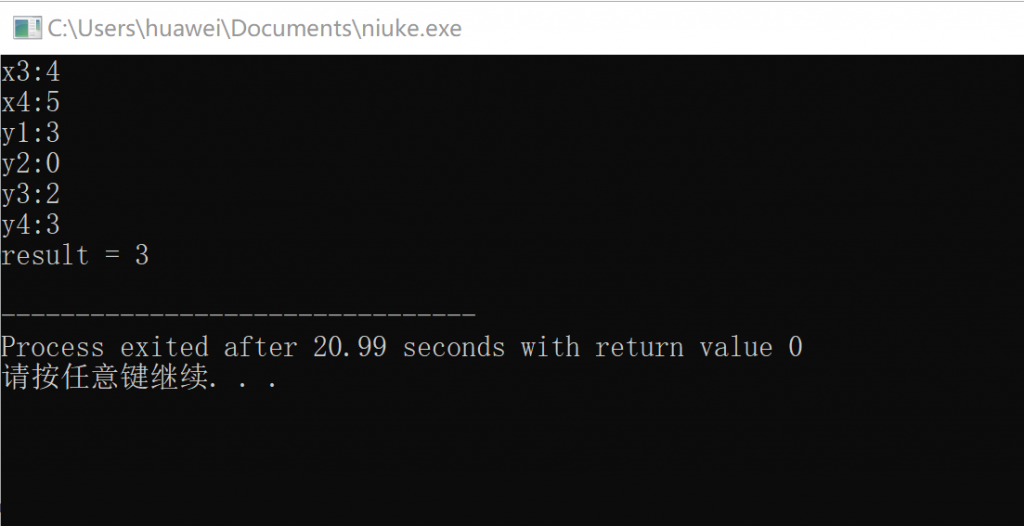发表于 2019-2-9 11:08:39 | 显示全部楼层
 hjwwwwww 发表于 2019-2-9 07:23 你好 但是结果是4哦 你的代码结果是3 我试了一下，答案是4呀E:\2.png发表于 2019-2-9 11:15:31 | 显示全部楼层
 #include int main() {         int N, i, j, y;         int temp = 0;         int tem = 0;         int result = {0};         int row, column;         int mat = {0};         printf("point count:");         scanf("%d", &N);         for (i = 0; i < N; i++)         {                 printf("x%d:", i+1);                 scanf("%d", &row[i]);         }         for (j = 0; j < N; j++)         {                 printf("y%d:", j+1);                 scanf("%d", &column[j]);         }         for (i = 0; i < N; i++)         {                 mat[row[i]][column[i]] = 1;         }         for (j = 0; j < 100; j++)         {                 for (int z = 0; z < j+1; z++)                 {                         y = (-1) * z + j;                         if (mat[z][y])                         {                                 result[j]++;                         }                 }         }                 for (i = 0; i < sizeof(result) / sizeof(int); i++)         {                 if (result[i] > temp)                 {                         temp = result[i];                 }                 result[i] = 0;         }                 for (i = 100, j = 0; i >= 0; i--, j++)         {                 for (int z = i, x = 0; x < j + 1; x++, z++)                 {                         y = z - i;                         if (mat[z][y])                         {                                 result[j]++;                         }                 }         }         for (i = 0; i < (sizeof(result) / sizeof(int)); i++)         {                 if (result[i] > tem)                 {                         tem = result[i];                 }         }         printf("result = %d\n", temp+tem > N? temp+tem-1:temp+tem);         return 0; }复制代码发表于 2019-2-9 11:16:40 | 显示全部楼层
 做了下判断，决绝了两条直线不在区域内相交的情况发表于 2019-2-9 20:22:22 From FishC Mobile | 显示全部楼层
 hjwwwwww 发表于 2019-2-8 17:05 @BngThea 身边暂无生产力工具，不方便测试，感觉你的思路和题意不太一致。 要求的是找两条线，而不是所有满足斜率的点的集合发表于 2019-2-19 23:52:36 | 显示全部楼层
 本帖最后由 前路 于 2019-2-20 00:18 编辑 #include int main(void){     int N,i,j,k,temp_x,temp_y,Slope_Result;     int Slope_Result_1 = 0;     int Slope_Result_2 = 0;     int x, y;     int result = {0};     int xy = {0};             scanf("%d", &N);       //输入点的总数 /*分别输入测试点的x和y坐标*/     for(i = 0; i < N; i++){//输入x的值         scanf("%d", &x[i]);     }     for(j = 0; j < N; j++){//输入y的值         scanf("%d", &y[j]);     }     for (i = 0; i < N; i++){//将输入的点显示出来         xy[ (x[i]) ][ (y[i]) ] = 1;     } /*计算斜率为-1时的所有直线上 存在输入点情况*/     for (i = 0; i < 100; i++){         for (temp_x = 0; temp_x < i+1; temp_x++){             temp_y = (-1) * temp_x + i;             if (xy[temp_x][temp_y]){                 result[i]++;             }         }     } /*计算出斜率为-1时直线上最多点的个数*/     for (i = 0; i < ( sizeof(result)/sizeof(int) ) ; i++){         if (result[i] > Slope_Result_1){             Slope_Result_1 = result[i];             }         result[i] = 0;     } /*计算斜率为1时的所有直线上 存在输入点情况*/     for (i = 100, j = 0 ; i >=0; i--, j++){         for (temp_x = i, k = 0 ; k < j + 1; temp_x++, k++){             temp_y = temp_x - i;             if (xy[temp_x][temp_y]){                 result[i]++;             }         }     } /*计算斜率为1时直线上最多点的个数*/     for (i = 0; i < ( sizeof(result)/sizeof(int) ) ; i++){         if (result[i] > Slope_Result_1){             Slope_Result_2 = result[i];             }         result[i] = 0;     } /*计算满足条件的点的总和*/     Slope_Result = Slope_Result_1 + Slope_Result_2;         printf("%d\n", Slope_Result > N ? Slope_Result-1 : Slope_Result);     return 0; }复制代码 感谢 12#楼鱼油 提供的思路 具体回复如下楼主| 发表于 2019-2-20 12:46:49 | 显示全部楼层
 前路 发表于 2019-2-19 23:52 感谢 12#楼鱼油 提供的思路 具体回复如下 原题链接：https://ac.nowcoder.com/acm/problem/18951 大佬可以再看一下吗？好像还是不对...但是悬赏先感恩啦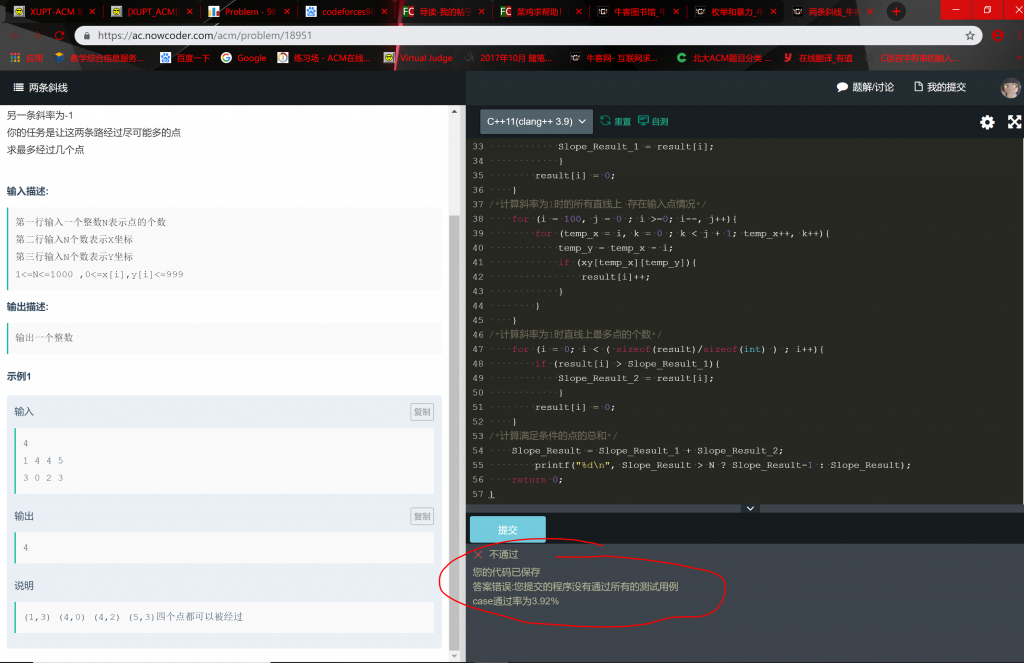发表于 2019-2-20 13:01:11 | 显示全部楼层
 hjwwwwww 发表于 2019-2-20 12:46 原题链接：https://ac.nowcoder.com/acm/problem/18951 大佬可以再看一下吗？好像还是不对...但是悬赏先 ... 好的我马上去测试一下！ 不过牛客网的编程题大多考虑必须全面，我以前也用，不过强迫症的我最后还是选择了搁置！

 本版积分规则 回帖后跳转到最后一页

## 小甲鱼强烈推荐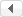/1GMT+8, 2019-8-25 04:42

Powered by Discuz! X3.4

© 2001-2017 Comsenz Inc.